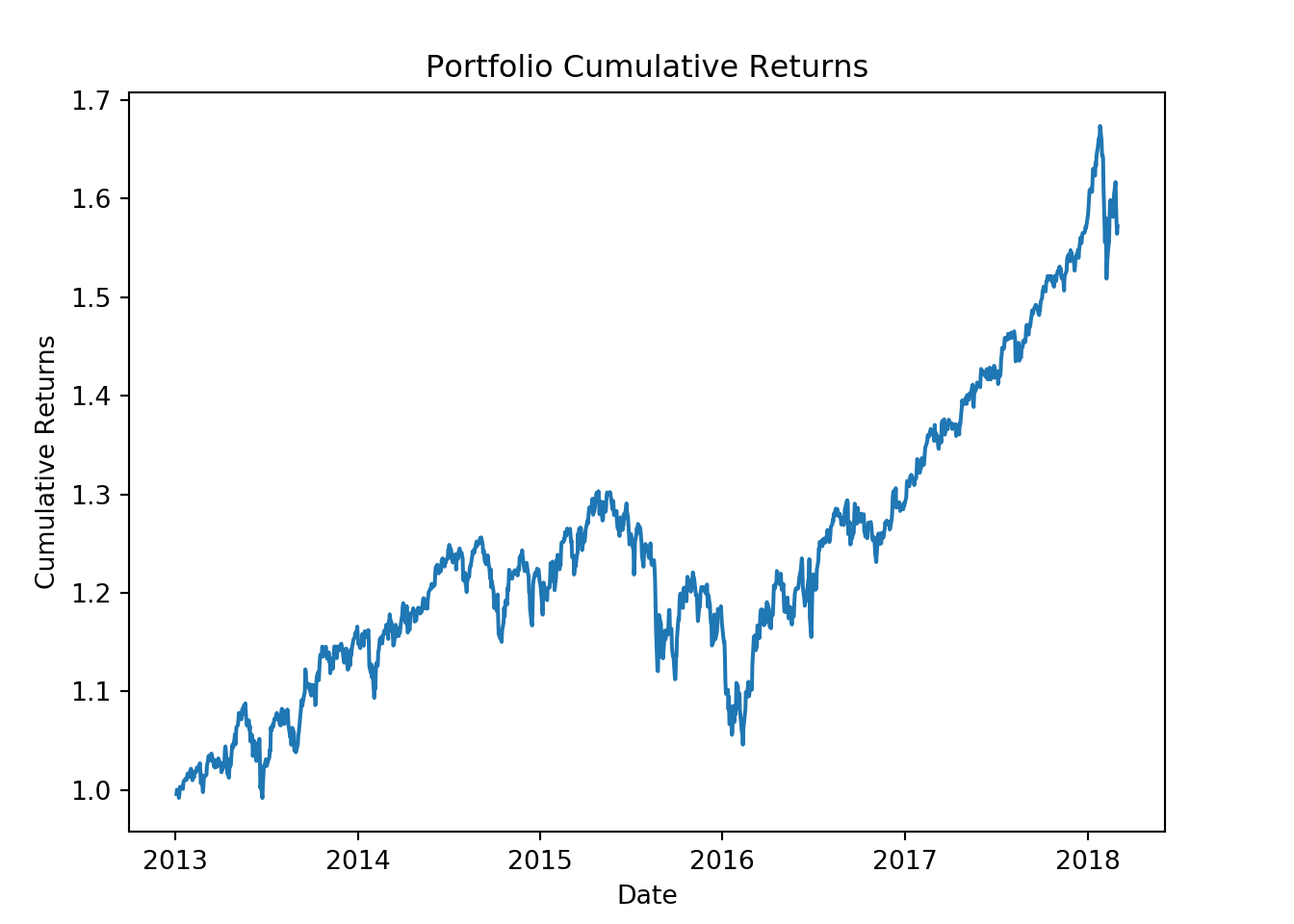In this post we will calculate the following portfolio statistics using Python.

• Portfolio average returns
• Portfolio standard deviation
• Portfolio Sharpe ratio

``````import pandas as pd
import numpy as np
import matplotlib.pyplot as plt
import pandas_datareader as web``````

We will use the same assets from the last post to build our portfolio.

``````# Create a list of tickers and weights
tickers = ['BND', 'VB', 'VEA', 'VOO', 'VWO']
wts = [0.1,0.2,0.25,0.25,0.2]``````

Next lets download the price data from yahoo finance. We will only keep the adjusted closing price for our stock tickers.

``````price_data = web.get_data_yahoo(tickers,
start = '2013-01-01',
end = '2018-03-01')
price_data = price_data['Adj Close']
``````

Next we will calculate the daily returns for our assets.

``ret_data = price_data.pct_change()[1:]``

After this we will calculate the portfolio returns.

``port_ret = (ret_data * wts).sum(axis = 1)``

Lets look at the total cumulative returns for our portfolio.

``````cumulative_ret = (port_ret + 1).cumprod()
fig = plt.figure()
ax1.plot(cumulative_ret)
ax1.set_xlabel('Date')
ax1.set_ylabel("Cumulative Returns")
ax1.set_title("Portfolio Cumulative Returns")
plt.show();``````We now have the daily returns data. We will convert it to geometric average annual returns.

``````geometric_port_return = np.prod(port_ret + 1) ** (252/port_ret.shape) - 1
print(geometric_port_return)``````
``## 0.09164978620705222``

Next we will calculate the portfolio standard deviation or volatility. This is the annual standard deviation for our portfolio.

``````annual_std = np.std(port_ret) * np.sqrt(252)
print(annual_std)``````
``## 0.12039589835434619``

Next we will calculate the portfolio Sharpe ratio.

``````port_sharpe_ratio = geometric_port_return / annual_std
print(port_sharpe_ratio)``````
``## 0.7612367818155304``

In this post we calculate the

• Portfolio annualized returns
• Portfolio standard deviation
• Portfolio Sharpe ratio